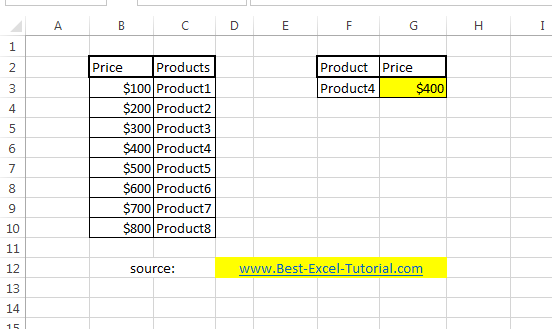#### How to Vlookup Backwards

Something tricky today. Let’s create some backwards VLOOKUP formula. It’s quite easy, but not obvious. Here’s an example.

Vlookup is a powerful function in Excel that can be used to search for a specific value in a range of cells and return a corresponding value from a different column in the same row. By default, Vlookup searches for values in the first column of a range and returns the corresponding value from a specified column. However, sometimes you may want to search for a value in a column to the right of the range and return a corresponding value from a column to the left.

## Sample dataset for Vlookup

The sample data table shows the products and prices. You try to find the price.## Backwards Vlookup formula

To perform a “Vlookup backwards” in Excel, you can use the INDEX and MATCH functions together. The INDEX function can return a value from a specific cell within a range of cells, while the MATCH function can search for a value within a range of cells and return its relative position. By combining these two functions, you can create a Vlookup-like function that works in reverse.

The formula of reverse VLOOKUP is: =INDEX(\$C\$3:\$C\$10,MATCH(\$F\$3,\$B\$3:\$B\$10,0))

Explanation:

C column – prices – array where you have your values whose you are looking for.

B column – products – names of all products which prices you know.

F3 – name of the product, which price you are looking for.

Now you know that you can also do a reverse vlookup.

This is a simple example of how to perform a Vlookup backwards in Excel. You can modify the formula and the data as needed for your specific requirements.